## Mathematics: Toolkit

### Algebra

The first problem in Michael's manuscript concerns the value of 315 pounds of pepper. After solving the problem by the rule of three, Michael says he will solve the problem again by "la cosa." Literally cosa means "thing." In Michael's time, it was used to refer to the "unknown thing" in an algebraic expression—the variable being sought—and to algebra as a whole.

Modern algebraic notation did not exist in Michael's time, so he wrote out the entire problem and its solution in words. This makes his solution difficult to follow at times, since there are no symbols or calculations to help us visualize his thought processes.

Michael begins by recalling one of the algebraic statements he included in the discussion of algebra that comes later in his manuscript; namely, if there is an "unknown equal to a number, the number should be divided by the unknown." In modern notation, this means that if you started with: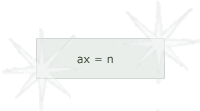the solution would be: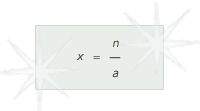Michael does indeed work the problem into the form of the first equation and then solve it by the second.

• a) The value in ducats

Michael begins by putting his problem in the proper order.

• 1] He first states: "let the value sought be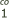," where "co" stands for cosa, or "unknown." In modern notation,would be x.

• 2] He then restates his original problem: "Ifgives me 315 pounds, what will give me 49ducats, which is?" This statement is a little puzzling because he already knows 400 pounds will get him 49ducats, but doesn't mention it. Subsequent calculations make it clear that he had in mind the following equation: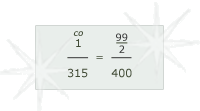• 3] In his mind, or on a piece of paper, Michael may have listed the figures: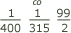.
• 4] Michael begins to operate on these numbers, multiplying 99 by 315 to get 31185.

• 5] Next he multiplies 400 by, to get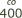(or 400x).

• 6] Then he divides 31185 by 2 to get 15592.

• 7] Now he has the problem in the form of ax = n, or: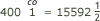.
• 8] All he has to do is divide 15592by 400. The result, he says, will be 38 ducats.

• b) The remainder in denari and piccoli

Michael simply reminds the reader to multiply the remainder by 24 and divide by 400 to get the denari and to repeat the process using 32 to get the piccoli. He doesn't actually do it.

He concludes that 315 pounds of pepper is worth 38 ducats, 23 denari, and 17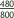piccoli. He stresses the fact that this is the same answer he got using the rule of three.

See how Michael solves the problem using the rule of three or the rule of double false position.